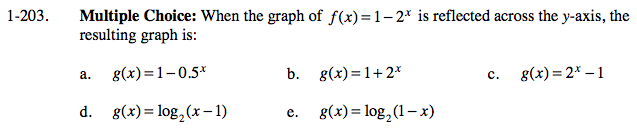### Home > CALC > Chapter 1 > Lesson 1.5.1 > Problem1-203

1-203.
1. Multiple Choice: When the graph of f(x) = 1 − 2x is reflected across the y-axis, the resulting graph is: Homework Help ✎

1. g(x) = 1 − 0.5x

2. g(x) = 1 + 2x

3. g(x) = 2x − 1

4. g(x) = log2(x − 1)

5. g(x) = log2(1 − x)Reflective symmetry across the y-axis means that f(x) is an even function. The definition of even functions is f(x) = f(−x).

Evaluate f(−x)...